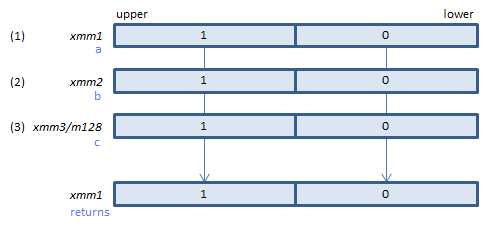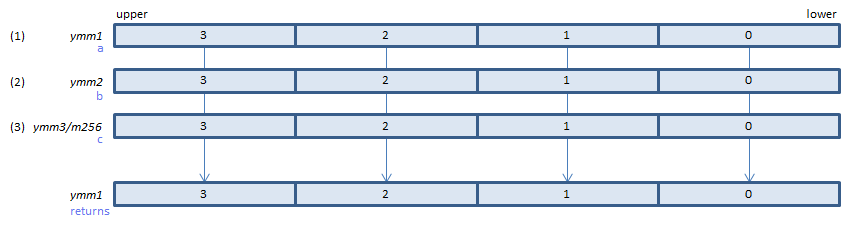﻿ vpternlogq

## VPTERNLOGQ - Packed TERNary LOGic Qword

VPTERNLOGQ xmm1{k1}{z}, xmm2, xmm3/m128/m64bcst, imm8    (V5+VL
__m128i _mm_ternarylogic_epi64(__m128i a, __m128i b, __m128i c, int imm8);For each bit, performs a ternary bitwise logical operation specified by imm8 and sets the result to the first operand. (returns the result.)
VPTERNLOGQ ymm1{k1}{z}, ymm2, ymm3/m256/m64bcst, imm8    (V5+VL
__m256i _mm256_ternarylogic_epi64(__m256i a, __m256i b, __m256i c, int imm8);For each bit, performs a ternary bitwise logical operation specified by imm8 and sets the result to the first operand. (returns the result.)
VPTERNLOGQ zmm1{k1}{z}, zmm2, zmm3/m512/m64bcst, imm8    (V5
__m512i _mm512_ternarylogic_epi64(__m512i a, __m512i b, __m512i c, int imm8);For each bit, performs a ternary bitwise logical operation specified by imm8 and sets the result to the first operand. (returns the result.)

imm8

Any logical operation can by specified by the following steps:

1. Make a logical expression using (1) (2) (3).
2. Assign (1) = F0, (2) = CC, (3) = AA, and calculate the expression to get a 8-bit result.
3. Specify the result of 2 for imm8.

Or make a table like below to find 8-bit to specify for imm8.

binary
(1) = F0 1 1 1 1 0 0 0 0
(2) = CC 1 1 0 0 1 1 0 0
(3) = AA 1 0 1 0 1 0 1 0
resulting 8-bit imm8

x86/x64 SIMD Instruction List  Feedback